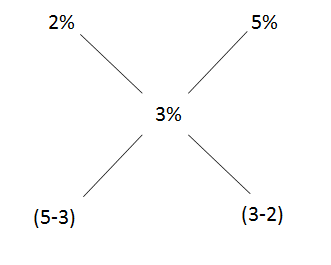# CAT Quantitative Aptitude Questions | CAT Ratios, Mixtures, Alligations and Averages Questions

###### CAT Questions | Alligations and Averages | Alligation

The question is from CAT Ratio, Mixtures and Averages. When a sum of amount is lend at two different percentages, what is the amount lend at one particular rate? CAT exam is known to test on basics rather than high funda ideas. A range of CAT questions can be asked from Ratios and Proportions, Mixtures, Alligations and Averages. Make sure you master the topics. 2IIMs CAT questions bank provides you with CAT questions that can help you gear for CAT Exam CAT 2019.

Question 29: Ram borrows Rs 4000 on simple interest from Shyam for a period of 4 years. He borrows a portion of amount at 2% interest and the remaining at 5%. If the interest Shyam earns is Rs 480, How much money did Ram borrow at 2% interest rate?

1. $$frac{8000}{3}\\$ 2. $\frac{4000}{3}\\$ 3. 3000 4. 2000 ## 2IIM : Best Online CAT Coaching #### 2IIM's Online CAT CoachingGet CAT Last Mile Prep Course for 799 /-CAT Online Coaching ## Best CAT Coaching in Chennai #### CAT Coaching in Chennai - CAT 2020Starts Sat, November 2nd, 2019 ### Explanatory Answer ##### Method of solving this CAT Question from Ratio, Mixtures and Averages: When we mix two mixtures in a particular ratio, we get a third mixture. Given the third mixture how does one find the ratio in which they were mixed. Interest earned per year = Rs.$\frac{480}{4}\\$ = Rs 120 Therefore, % earned per year = $\frac{120}{4000}\\$ = 3%So, amount invested in 2% = $\frac{2}{3}\\$ * 4000 = $\frac{8000}{3}\\$ The question is "How much money did Ram borrow t 2% interest rate?" ##### Hence, the answer is "$\frac{8000}{3}\\$". Choice A is the correct answer. ###### 2IIM's Online CAT CoachingGet CAT Last Mile Prep Course for 799 /- Signup Now! ###### Already have an Account? ###### CAT Coaching in ChennaiCAT 2020Enroll at 44,000/- Next Weekend Batch Starts Sat, Nov 2nd, 2019 ###### Best CAT Coaching in ChennaiRegister Online, get Rs 4000/- off Attend a Demo Class ##### Where is 2IIM located? 2IIM Online CAT Coaching A Fermat Education Initiative, 58/16, Indira Gandhi Street, Kaveri Rangan Nagar, Saligramam, Chennai 600 093 ##### How to reach 2IIM? Phone:$91) 44 4505 8484
Mobile: (91) 99626 48484
WhatsApp: WhatsApp Now
Email: prep@2iim.com